# GMAT Math : DSQ: Calculating circumference

## Example Questions

### Example Question #34 : Radius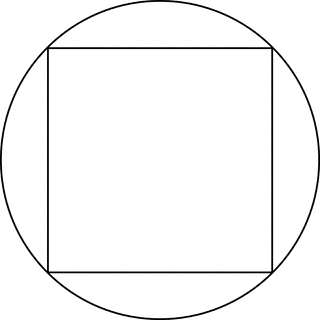Square S is inscribed in circle C as in the figure above. What is the circumference of C?

(1) The perimeter of S is 16.

(2) The area of S is 36.

C. BOTH statements TOGETHER are sufficient, but NEITHER statement ALONE is sufficient.

E. Statements (1) and (2) TOGETHER are NOT sufficient.

A. Statement (1) ALONE is sufficient, but statement (2) alone is not sufficient.

B. Statement (2) ALONE is sufficient, but statement (1) alone is not sufficient.

D. EACH statement ALONE is sufficient.

D. EACH statement ALONE is sufficient.

Explanation:

From statement (1), we know that the side of S is 4, and then we can calculate the diagonal of S using the Pythagorean theorem: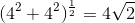. The diagonal of S is the diameter of C. Therefore, we can calculate the circumference by using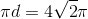. From statement (2), we know that the side of S is 6, and then we can calculate the diagonal of S using the Pythagorean theorem: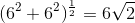The diagonal of S is the diameter of C. Therefore, we can calculate the circumference by using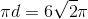.

### Example Question #581 : Data Sufficiency Questions

What is the circumference of circle J?

I) Circle J has an area of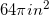.

II) Circle J has a diameter of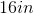.

Statement 2 is sufficient to solve the question, but statement 1 is not sufficient to solve the question.

Statement 1 is sufficient to solve the question, but statement 2 is not sufficient to solve the question.

Both statements taken together are sufficient to solve the question.

Neither statement is sufficient to solve the question. More information is needed.

Each statement alone is enough to solve the question.

Each statement alone is enough to solve the question.

Explanation:

We are given the area and diameter of a circle and asked to find the circumference. We know that diameter is twice the length of a radius, so we also have our radius.

Given the following equations: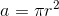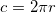We can see that knowing either diameter or area will allow us to find the circumference.

Thus: Each statement alone is enough to solve the question.

### Example Question #36 : Radius

What is the circumference of the circle given by: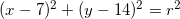I)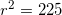.

II) The slope of the tangent to the circle at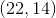is undefined.

Statement II is sufficient to answer the question, but Statement I is not sufficient to answer the question.

Both statements together are needed to answer the question.

Statement I is sufficient to answer the question, but Statement II is not sufficient to answer the question.

Either statement alone is sufficient to answer the question.

Either statement alone is sufficient to answer the question.

Explanation:

All we need to find circumference is the radius.

I) Gives us the radius squared, so we could find circumference with I.

II) Tells us the slope of the tangent line at a given point is undefined. Only vertical lines have undefined slope. The tangent line is perpendicular to the radius, so we can find our radius by drawing a picture and comparing the location of the center to the location of the tangent line.

So either statement will be sufficient.

### Example Question #37 : Radius

What is the circumference of Circle?

1.) The radius of the circle is.

2.) The circle is inside another circle of area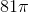.

Statement 1 is sufficient to solve the question, but Statement 2 is not sufficient to solve the question.

Each statement alone is sufficient to solve the question.

Both statements taken together are sufficient to solve the question.

Statement 2 is sufficient to solve the question, but Statement 1 is not sufficient to solve the question.

Neither statement is sufficient to solve the question. More information is needed.

Statement 1 is sufficient to solve the question, but Statement 2 is not sufficient to solve the question.

Explanation:

We are asked to find the circumferenceof Circleand are given the diameter and the area. We also know that.  Taking each statement individually:

1.) The radiusisand we know that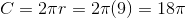. Therefore, Statement 1 is sufficient to solve for the circumference of the circle by itself.

2.) The areaof the outside circle is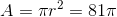, but we cannot use this to determine the circumference of Circlebecause we don't know whereis inside the larger outside circle.

### Example Question #38 : Radius

What is the circumference of Circle?

1.) The diameter of the circle is.

2.) The area of the circle is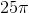.

Each statement alone is sufficient to solve the question.

Both statements taken together are sufficient to solve the question.

Neither statement is sufficient to solve the question. More information is needed.

Statement 1 is sufficient to solve the question, but Statement 2 is not sufficient to solve the question.

Statement 2 is sufficient to solve the question, but Statement 1 is not sufficient to solve the question.

Each statement alone is sufficient to solve the question.

Explanation:

We are asked to find the circumferenceof Circleand are given the diameter and the area. We also know that.  Taking each statement individually:

1.) The diameterisand we know that the radius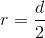, so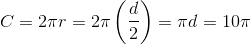. Therefore, Statement 1 is sufficient to solve for the circumference of the circle by itself.

2.) The areaof Circleis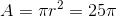, so we can determine that the radius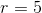. Since the circumference, Statement 2 is is sufficient to solve for the circumference of the circle by itself.

Tired of practice problems?

Try live online GMAT prep today.